Author: Oscar Cronquist Article last updated on May 05, 2022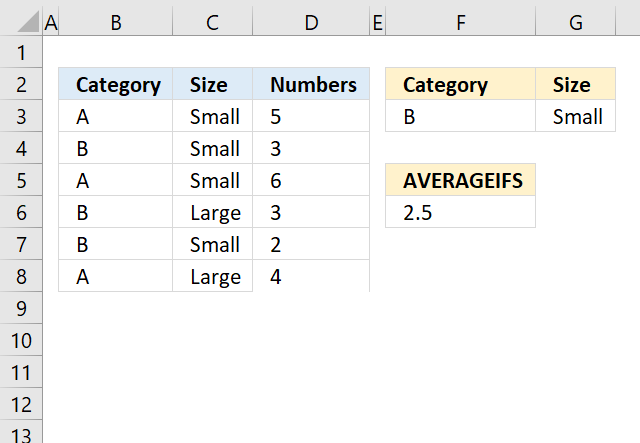The AVERAGEIFS function returns the average of cell values that evaluates to TRUE for multiple criteria.

Formula in cell F6:

=AVERAGEIFS(D3:D8,B3:B8,F3,C3:C8,G3)

## 1. AVERAGEIFS Function Syntax

AVERAGEIFS(average_range, criteria_range1, criteria1, [criteria_range2] , [criteria2], ...)

The AVERAGEIFS function allows you to have up to 127 criteria and criteria_range arguments.

## 2. AVERAGEIFS Function Arguments

 average_range Required. One or more cells to average, including numbers or names, arrays, or references that contain numbers. criteria_range1 Required. A cell range in which to evaluate the corresponding condition criteria1 Required.  A condition in the form of a cell reference, expression or text that determines which values to be averaged. [criteria_range2] Optional. You are allowed to have multiple criteria ranges. [criteria2] Optional. You are allowed to have multiple criteria.

## 3. What values are excluded?

Boolean values TRUE or FALSE are excluded in the calculation.

An empty cell in argument average_range is excluded.

## 4. AVERAGEIFS function not working

A blank or text value in the argument range is not valid, the function returns #DIV0!.

A value is used in the calculation only if all of the corresponding criteria specified are true for that cell.

If a condition in argument average_range is empty is the same as 0 (zero).

The AVERAGEIFS function returns #DIV0! if no values match the criteria.

## 5. AVERAGEIFS Function example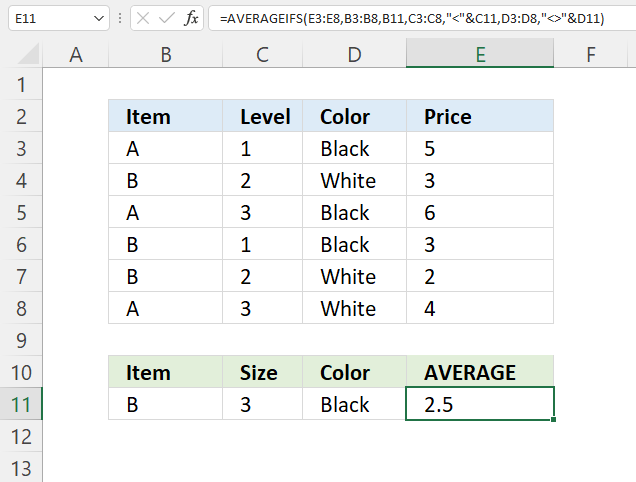Formula in cell F6:

AVERAGEIFS(E3:E8,B3:B8,B11,C3:C8,"<"&C11,D3:D8,"<>"&D11)

The formula in cell F6 in the picture above calculates the average for values that are in

### Explaining formula

The AVERAGEIFS function lets you use comparison operators, they are:

• < less than character
• > larger than character
• <= less than or equal to
• >= larger than or equal to
• <> not equal to

#### Step 1 - AVERAGEIFS function

AVERAGEIFS(average_range, criteria_range1, criteria1, [criteria_range2] , [criteria2], ...)

#### Step 2 - Populate arguments

average_range - E3:E8
criteria_range1 - B3:B8
criteria1 - B11
criteria_range2 - C3:C8
criteria2 - "<"&C11
criteria_range3 - D3:D8
criteria3 - "<>"&D11

#### Step 3 - Evaluate function

AVERAGEIFS(D3:D8, B3:B8, F3, C3:C8, G3)

becomes

AVERAGEIFS({5; 3; 6; 3; 2; 4}, {"A"; "B"; "A"; "B"; "B"; "A"}, "B", {1; 2; 3; 1; 2; 3}, "<"&3, {"Black"; "White"; "Black"; "Black"; "White"; "White"}, "<>"&"Black")

and returns 2.5

First condition matches cells B4,B6, and B7.
The second condition matches C3, C4, C6, and C7.
The third condition matches D4, D7, and D8.

Rows that match all conditions are 4 and 7, cells E4 and E7 contain 3 and 2. 3 + 2 equals 5. 5/2 is 2.5

## 6. AVERAGEIFS Function - wildcard characters

It is possible to use wildcard characters like (?) question mark or (*) asterisk.

• (?) question mark matches any single character.
• (*) asterisk matches any sequence of characters.
• Use a tilde (~) before to escape the wildcard characters meaning finding the actual question mark or asterisk.

## 6.1 AVERAGEIFS Function - begins with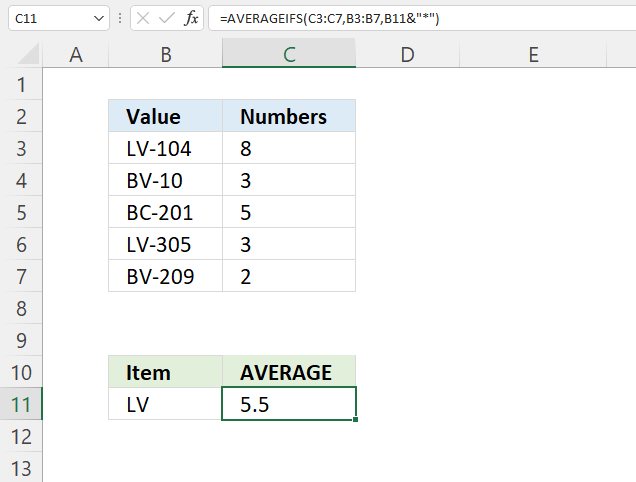The image above demonstrates how to average numbers if values on the same row begin with a specific condition.

Formula in cell F3:

=AVERAGEIFS(C3:C7, B3:B7, B11&"*")

### Explaining formula

#### Step 1 - Populate arguments

AVERAGEIFS(average_range, criteria_range1, criteria1, [criteria_range2] , [criteria2], ...)

average_range - B3:B7
criteria - E3&"*"
criteria_range - C3:C7

#### Step 2 - Evaluate AVERAGEIF function

AVERAGEIFS(C3:C7, B3:B7, B11&"*")

becomes

AVERAGEIFS({8; 3; 5; 3; 2}, {"LV-104"; "BV-10"; "BC-201"; "LV-305"; "BV-209"}, "LV*")

and returns 5.5

Cells B3 and B6 begin with the given value in cell B11, the corresponding values are in C3 and C6. They contain 8 and 3, 8 + 3 equals 11. 11/2 equals 5.5

## 6.2 AVERAGEIFS Function - ends with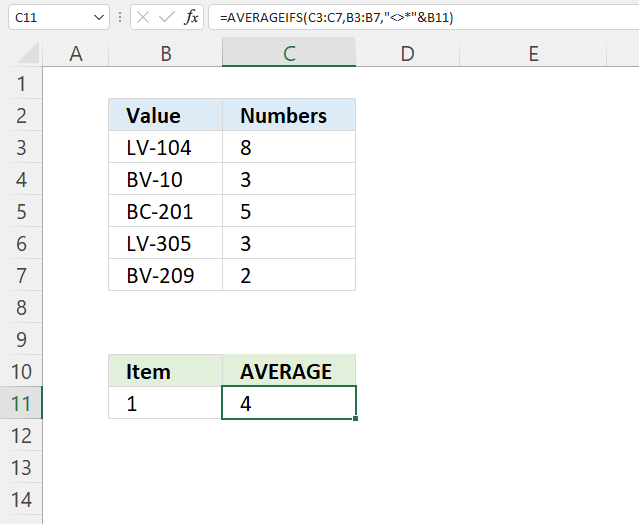The formula in cell C11 calculates an average based on numbers in C3:C7 if the corresponding value in cell range B3:B7 does NOT end with the value specified in cell B, in this example, 1.

Cells B3, B4, B6, and B7 contain values that don't end with 1, the corresponding numbers on the same rows are 8, 3, 3, and 2. 8 + 3 + 3 + 2 equals 16. 16/4 equals 4

Formula in cell C11:

=AVERAGEIFS(C3:C7,B3:B7,"<>*"&B11)

### Explaining formula

#### Step 1 - Populate arguments

AVERAGEIFS(average_range, criteria_range1, criteria1, [criteria_range2] , [criteria2], ...)

average_range - B3:B7
criteria - "<>*"&B11
criteria_range - C3:C7

#### Step 2 - Evaluate AVERAGEIF function

AVERAGEIFS(C3:C7, B3:B7, "<>*"&B11)

becomes

AVERAGEIFS({8; 3; 5; 3; 2}, {"LV-104"; "BV-10"; "BC-201"; "LV-305"; "BV-209"}, "<>*1")

and returns 4.

## 6.3 AVERAGEIFS Function - contains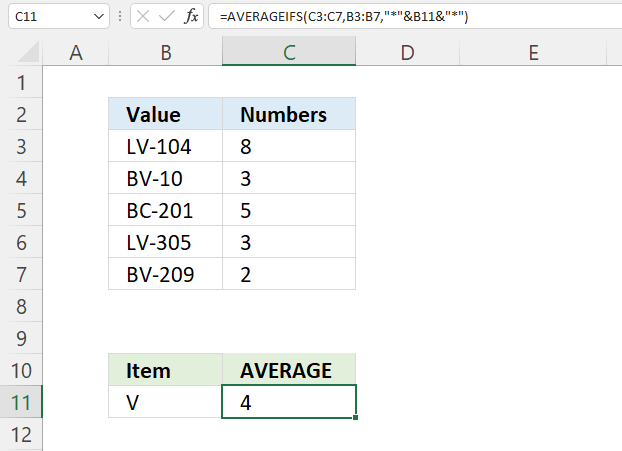The AVERAGIFS function in cell C11 calculates an average based on numbers in cell range C3:C7 if the corresponding value on the same row in cell range B3:B7 contains the condition specified in cell B11.

Formula in cell C11:

=AVERAGEIFS(C3:C7, B3:B7, "*"&B11&"*")

### Explaining formula

#### Step 1 - Populate arguments

AVERAGEIFS(average_range, criteria_range1, criteria1, [criteria_range2] , [criteria2], ...)

average_range - C3:C7
criteria_range1 - B3:B7
criteria - "*"&B11&"*"

#### Step 2 - Evaluate AVERAGEIF function

AVERAGEIFS(C3:C7, B3:B7, "<>*"&B11)

becomes

AVERAGEIFS({8; 3; 5; 3; 2}, {"LV-104"; "BV-10"; "BC-201"; "LV-305"; "BV-209"}, "*V*")

and returns 4.

## 7. AVERAGEIFS Function - nested functions and arrays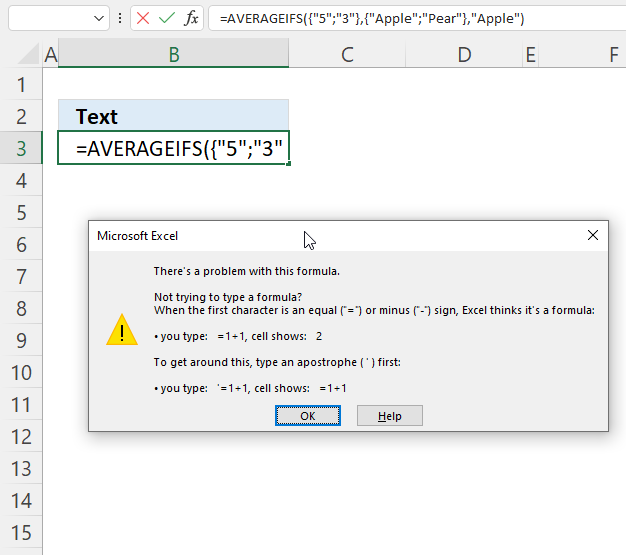The AVERAGEIFS function will not accept arrays or nested functions in any of the arguments which makes the AVERAGEIFS function not that useful in my opinion.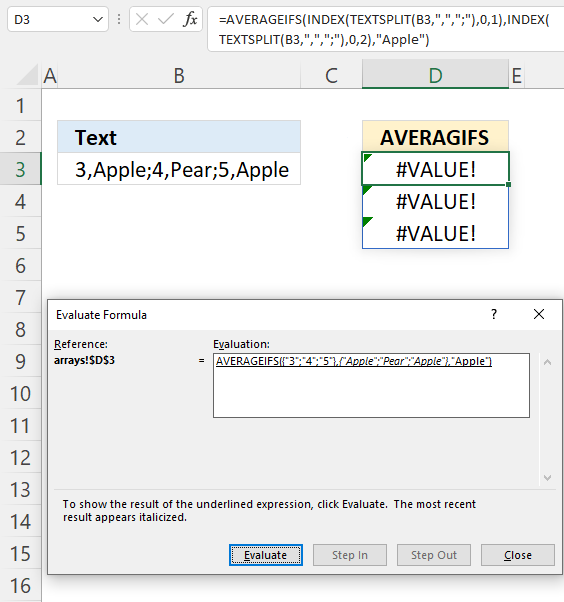The image above demonstrates the AVERAGEIFS function nested with the TEXTSPLIT and INDEX function, unfortunately, it doesn't work.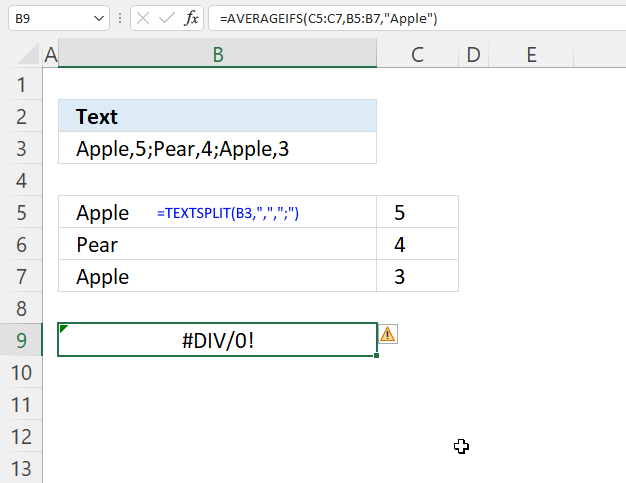What if we use "helper"-cells to perform intermediate calculations? Cell B5 contains: TEXTSPLIT(B3, ",", ";"). It is a dynamic array formula that spills values to cells below and to the right based on delimiting characters.

No, the AVERAGEIFS function returns a #DIV/0! error. The AVERAGEIFS function seems to be incompatible with new dynamic array functions in Excel 365.

Referencing dynamic arrays won't work either:

=AVERAGEIFS(INDEX(B5#,0,2),INDEX(B5#,0,1),"Apple")

This returns a #DIV/0! error as well.